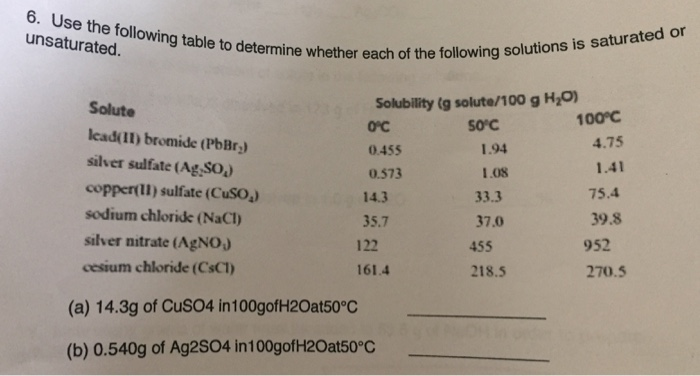# Question & Answer: se the following table to determine whether each of the following solution is saturated or…..Use the following table to determine whether each of the following solution is saturated or unsaturated. (a) 14.3g of CuSO4 in 100 g of H_2O at 50 degree C _____ (b) 0.540g of Ag2SO4 in100 g of H_2O at 50 degree C _____

a)

basis is 100 g of water, so we can compare directly

Don't use plagiarized sources. Get Your Custom Essay on
Question & Answer: se the following table to determine whether each of the following solution is saturated or…..
GET AN ESSAY WRITTEN FOR YOU FROM AS LOW AS \$13/PAGE

for CuSO4, in the data:

33.3 g of CuSO4 are soluble at 50 ºC

we have only 14.3 g of CuSO4, therefore, this is under the saturation point

choose unsaturated solution

b)

similar, we have the same basis so

saturation of Ag2SO4 = 1.08 g

we have only 0.54 g

therefore,

this is under the saturation point

choose unsaturated solution